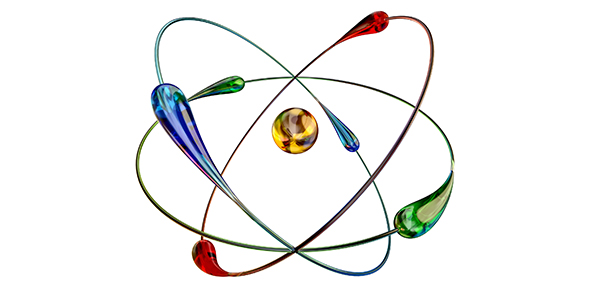# 3.1 Quiz - Nuclear Emissions

Approved & Edited by ProProfs Editorial Team
At ProProfs Quizzes, our dedicated in-house team of experts takes pride in their work. With a sharp eye for detail, they meticulously review each quiz. This ensures that every quiz, taken by over 100 million users, meets our standards of accuracy, clarity, and engagement.
| Written by Regentschemistry
R
Regentschemistry
Community Contributor
Total Contribution - 8 | Total attempts - 21,052
Questions: 10 | Attempts: 407SettingsDo this quiz ONLY with your Reference Tables!

• 1.

### The nucleus of U-238 is unstable. This causes U-238 to spontaneously...

• A.

Oxidize

• B.

Decay

• C.

Reduce

• D.

Combine

B. Decay
Explanation
Unstable nuclei are due to the neutron-to-proton ratio being greater than 1.5:1, causing the nucleus to spontaneously decay or break down.

Rate this question:

• 2.

### Which nuclear emission has the most mass and least penetrating power?

• A.

• B.

Alpha particles

• C.

Beta particles

• D.

Positrons

B. Alpha particles
Explanation
Alpha particles have the greatest mass of 4 amu (top number on Table O), but the least penetrating power (remember: alphabetical order... Alpha, Beta, Gamma).

Rate this question:

• 3.

### Which nuclear decay has the greatest penetrating power and the least mass?

• A.

Alpha

• B.

Beta

• C.

Gamma

• D.

Positron

C. Gamma
Explanation
Gamma radiation has the greatest penetrating power (remember: alpha, beta, gamma), but the least mass (0 amu, top number on Table O).

Rate this question:

• 4.

### Which nuclear decay consists of energy, only?

• A.

Gamma

• B.

Alpha

• C.

Beta

• D.

Positrons

A. Gamma
Explanation
Gamma particles are equivalent to energy. They have a mass of 0 amu (top number, Table O) and a charge of 0 (bottom number, Table O).

Rate this question:

• 5.

### Which nuclear decay consists of electrons?

• A.

Positrons

• B.

Gamma particles

• C.

Alpha particles

• D.

Beta particles

D. Beta particles
Explanation
Beta particles equal electrons. They have a mass of 0 amu (top number, Table O) and a charge of -1 (bottom number, Table O).

Rate this question:

• 6.

### Which nuclear decay consists of helium nuclei?

• A.

Positrons

• B.

Alpha particles

• C.

Beta particles

• D.

B. Alpha particles
Explanation
Alpha particles equal helium nuclei. They have a mass of 4 amu (top number, Table O) and a charge of +2 (bottom number, Table O).

Rate this question:

• 7.

### Which list correctly ranks nuclear emissions in order of increasing mass?

• A.

Alpha, beta, gamma, positron

• B.

Beta/Gamma/Positron, Alpha

• C.

Beta, Alpha, Gamma, Positron

• D.

Positron, Alpha, Gamma, Beta

B. Beta/Gamma/Positron, Alpha
Explanation
Beta, Gamma, and Positrons all have a mass of 0 amu (top number on Table O), meaning smallest mass. Alpha particles have the largest mass of 4 amu (top number on Table O).

Rate this question:

• 8.

### Which list correctly organizes nuclear emissions in order of increasing charge?

• A.

Beta, Positron, Gamma, Alpha

• B.

Positron, Beta, Gamma, Alpha

• C.

Beta, Gamma, Positron, Alpha

• D.

Alpha, Beta, Gamma, Positron

C. Beta, Gamma, Positron, Alpha
Explanation
Beta particles have the smallest charge of -1, Gamma particles have a charge of 0, Positrons have a charge of +1, Alpha particles have a charge of +2 (bottom number, Table O).

Rate this question:

• 9.

### Without looking at a graph, why is O-16 a stable nuclide?

• A.

Because it has a 8:1 ratio of protons to neutrons

• B.

Because it has a 1:1 neutron-to-proton ratio

• C.

Because it has a 1.6:1 neutron-to-proton ratio

• D.

Because it has a 16:8 proton-to-neutron ratio

B. Because it has a 1:1 neutron-to-proton ratio
Explanation
The number of protons equals atomic number. The number of neutrons equals mass number (16 for O-16) minus atomic number (8 for O-16). The band of stability (range of stabilities for neutron-to-proton ratios) is 1:1-1.5:1. Any nuclide with a neutron-to-proton ratio in this range is stable and will not spontaneously decay.

Rate this question:

• 10.

### Which of the following correctly lists the number of protons, the number of neutrons, the mass number, and the nuclide notation for N on this graph? (ONLY use this graph. Do not look at other references. I want you to test how much you know!!!!)

• A.

9 protons, 7 neutrons, Mass Number of 16, N-16 for nuclide

• B.

6 protons, 6 neutrons, Mass Number of 12, C-12 for nuclide

• C.

16 protons, 16 neutrons, Mass Number of 32, S-32 for nuclide

• D.

7 protons, 9 neutrons, Mass Number of 16, N-16 for nuclideBack to top